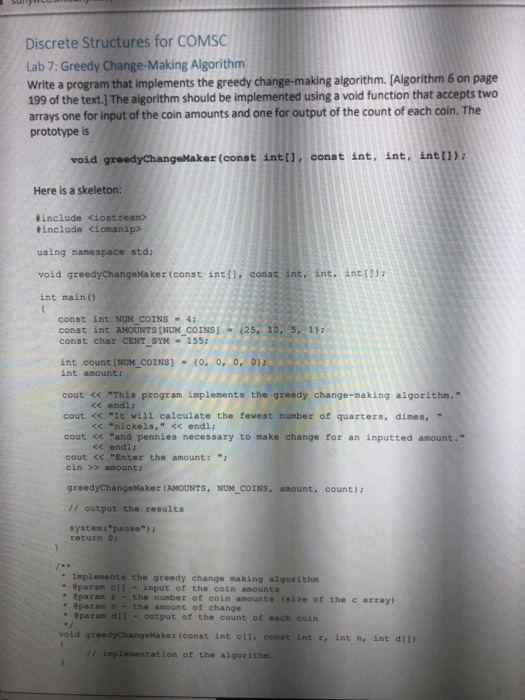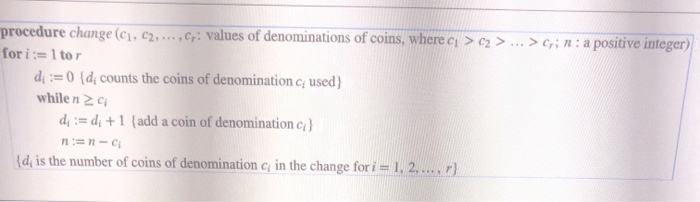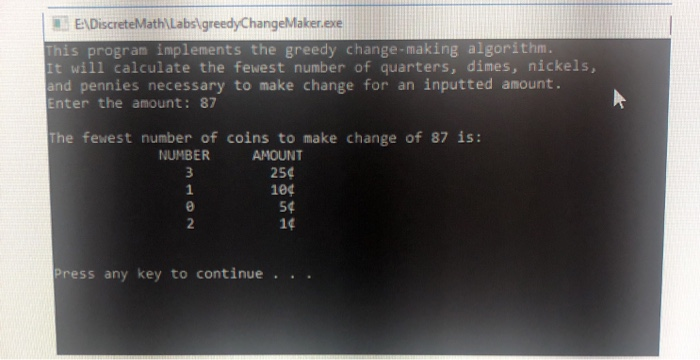# (Solved) : Write C Program Implements Greedy Change Making Algorithm Algorithm Output Look Like Q42710867 . . .

write a c++ program that implements the greedy change makingalgorithmalgorithmwhat the output should look likeDiscrete Structures for COMSC Lab 7: Greedy Change-Making Algorithm Write a program that implements the greedy change-making algorithm. [Algorithm 6 on page 199 of the text.] The algorithm should be implemented using a void function that accepts two arrays one for input of the coin amounts and one for output of the count of each coin. The prototype is void greedy ChangeMaker (const int(1,const int, int, int[]); Here is a skeleton: #include <iostream> #include <iomanip> using namespace std; void greedyChangeMaker (const int[], const int, int, int!) int main() const int NUM COINS 4 const int AMOUNTS (NUM COINS const char CENT SYM – 155: – (25, 10, 5, 1); int count (NUM_COINS) – 0, 0, 0, 0); int amount: cout << “This program implements the greedy change-making algorithm.” << endl; cout << “It will calculate the fewest number of quarters, dimes, << “nickels,” << endl; cout << “and pennies necessary to make change for an inputted amount.” << endl; cout << “Enter the amount : “; cin >> amount: greedyChangeMaker (AMOUNTS, NUM COINS, amount, count); // output the results system pause”) return 0 – Implements the greedy change making algorithm • @param – input of the coin amounta param – the number of coin amounts (size of the • @param n the amount of change @paramd -output of the count of each coin array) void greedyChangeMaker (const int al. const int r, int n, int dll) 11 implementation of the algorithm. procedure change (C1, C2, …,C: values of denominations of coins, where ci > C2 > … >Cin: a positive integer) for i:=1 tor di := 0 {di counts the coins of denomination cused) whileno di := d; +1 {add a coin of denomination c] n=n-C {d, is the number of coins of denomination in the change for i = 1, 2,…, r} EDiscrete MathLabs greedyChangeMaker.exe This program implements the greedy change-making algorithm. It will calculate the fewest number of quarters, dimes, nickels, and pennies necessary to make change for an inputted amount. Enter the amount: 87 The fewest number of coins to make change of 87 is: NUMBER AMOUNT 250 100 Press any key to continue … Show transcribed image text Discrete Structures for COMSC Lab 7: Greedy Change-Making Algorithm Write a program that implements the greedy change-making algorithm. [Algorithm 6 on page 199 of the text.] The algorithm should be implemented using a void function that accepts two arrays one for input of the coin amounts and one for output of the count of each coin. The prototype is void greedy ChangeMaker (const int(1,const int, int, int[]); Here is a skeleton: #include #include using namespace std; void greedyChangeMaker (const int[], const int, int, int!) int main() const int NUM COINS 4 const int AMOUNTS (NUM COINS const char CENT SYM – 155: – (25, 10, 5, 1); int count (NUM_COINS) – 0, 0, 0, 0); int amount: cout

Answer to write a c++ program that implements the greedy change making algorithm algorithm what the output should look like …

We are the best freelance writing portal. Looking for online writing, editing or proofreading jobs? We have plenty of writing assignments to handle.